Rheostat The schematic symbol for the rheostat is shown in Figure 10-57.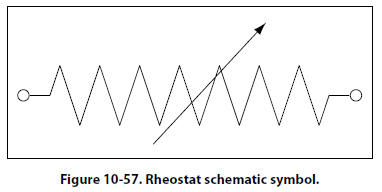A rheostat is a variable resistor used to vary the amount of current flowing in a circuit. Figure 10-58 shows a rheostat connected in series with an ordinary resistance in a series circuit.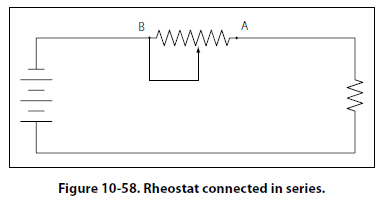As the slider arm moves from point A to B, the amount of rheostat resistance (AB) is increased. Since the rheostat resistance and the fixed resistance are in series, the total resistance in the circuit also increases, and the current in the circuit decreases. On the other hand, if the slider arm is moved toward point A, the total resistance decreases and the current in the circuit increases. Potentiometer The schematic symbol for the potentiometer is shown in Figure 10-59. The potentiometer is considered a three terminal device. As illustrated, terminals 1 and 2 have the entire value of the potentiometer resistance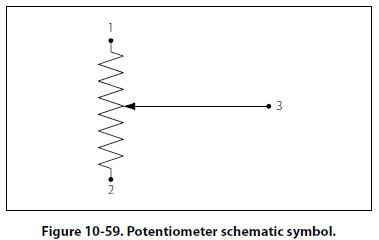between them. Terminal 3 is the wiper or moving contact. Through this wiper, the resistance between terminals 1 and 3 or terminals 2 and 3 can be varied. While the rheostat is used to vary the current in a circuit, the potentiometer is used to vary the voltage in a circuit. A typical use for this component can be found in the volume controls on an audio panel and input devices for flight data recorders, among many other applications. In Figure 10-60A, a potentiometer is used to obtain a variable voltage from a fixed voltage source to apply to an electrical load. The voltage applied to the load is the voltage between points 2 and 3. When the slider arm is moved to point 1, the entire voltage is applied to the electrical device (load); when the arm is moved to point 3, the voltage applied to the load is zero. The potentiometer makes possible the application of any voltage between zero and full voltage to the load.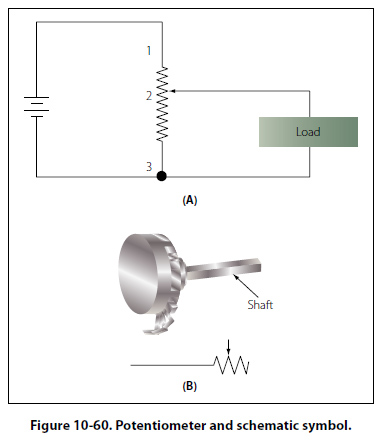The current flowing through the circuit of Figure 10-60 leaves the negative terminal electron flow of the battery and divides, one part flowing through the lower portion of the potentiometer (points 3 to 2) and the other part through the load. Both parts combine at point 2 and flow through the upper portion of the potentiometer (points 2 to 1) back to the positive terminal of the battery. In View B of Figure 10-60, a potentiometer and its schematic symbol are shown. In choosing a potentiometer resistance, the amount of current drawn by the load should be considered as well as the current flow through the potentiometer at all settings of the slider arm. The energy of the current through the potentiometer is dissipated in the form of heat. It is important to keep this wasted current as small as possible by making the resistance of the potentiometer as large as practicable. In most cases, the resistance of the potentiometer can be several times the resistance of the load. Figure 10-61 shows how a potentiometer can be wired to function as a rheostat.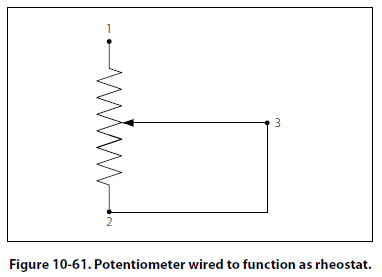©AvStop Online Magazine                                                                                                                                                      Contact Us              Return To Books